Author: Oscar Cronquist Article last updated on June 05, 2023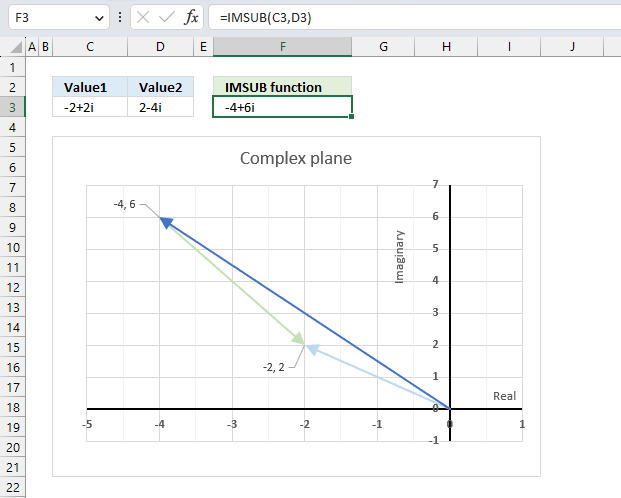What is the IMSUB function?

The IMSUB function calculates the difference between two complex numbers in x + yi or x + yj text format.

The letter j is used in electrical engineering to distinguish between the imaginary value and the electric current.

## 1. IMSUB Function Syntax

IMSUB(inumber1, inumber2)

## 2. IMSUB Function Arguments

 inumber1 Required. A complex number in x+yi or x+yj text format. inumber2 Required. A complex number in x+yi or x+yj text format.

## 3. IMSUB function example - rectangular form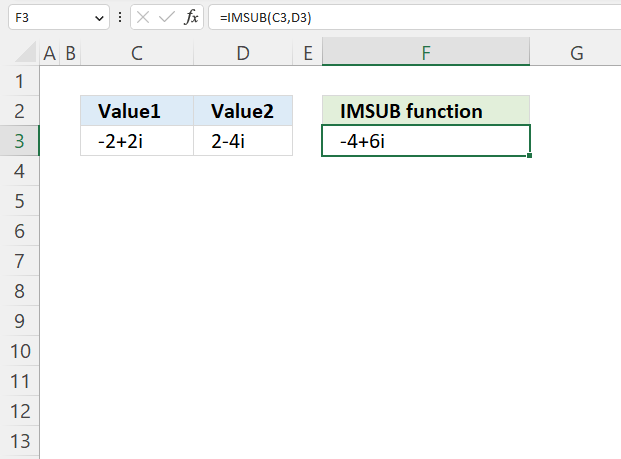The image above demonstrates the IMSUB function in cell F3 that calculates the difference between two complex numbers specified in cells C3 and D3.

Formula in cell F3:

=IMSUB(C3, D3)

### 3.1 Explaining formula

#### Step 1 - Populate arguments

IMSUB(inumber1, inumber2)

becomes

IMSUB(C3, D3)

#### Step 2 - Evaluate the IMSUB function

IMSUB(C3, D3)

becomes

IMSUB("-2+2i","2-4i")

and returns

-4+6i

## 4. How to calculate the difference between two complex numbers in rectangular form?This example demonstrates how Excel calculates in detail the difference between two complex numbers in rectangular form.

C1 is the first complex number specified in cell C3, C2 is the second complex number specified in cell D3.

C1 = x + yi

C2 = z + wi

To calculate the difference between two complex numbers we need to find the difference between the real numbers and the imaginary numbers separately.

C1- C2 = (x + yi) - (z + wi) = (x - z) + (y - w)i

C1 = -2+2i

C2 = 2-4i

C1- C2 = (-2 + 2i) - (2 - 4i) = ((-2) -2) + (2 - (-4))i  = -4+6i

## 5. How to graph the difference between two complex numbers?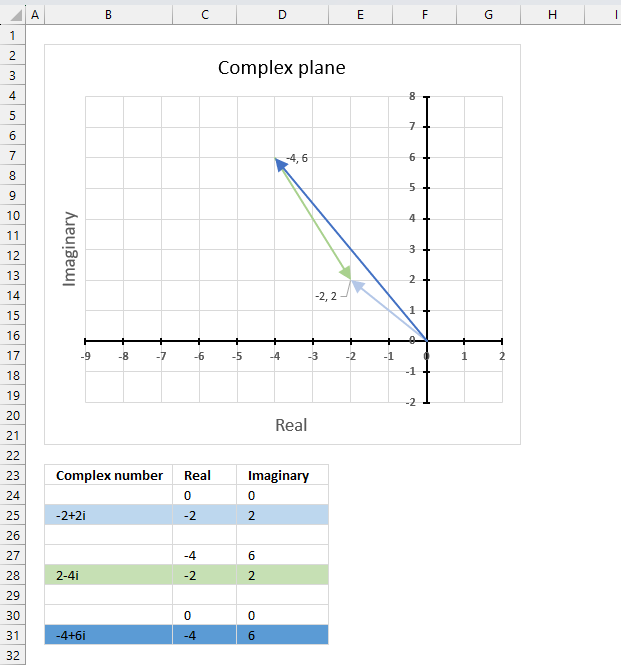The image above demonstrates a scatter chart that shows two different complex numbers and their difference, in total three different complex values.

Complex numbers in rectangular form a+bi have real and imaginary values which are positive, negative or zero, this makes it possible to use a chart with two dimensions x and y.

The imaginary values on the vertical axis (y) and the real values on the horizontal axis (x).

C1 = -2+2i (light blue)

C2 = 2-4i (green)

C1- C2  = -4+6i (dark blue)

Subtracting a complex number means that it points the opposite way with the same distance (green). The dark blue complex number is the difference between C1 and C2 .

### 5.1 Extract real and imaginary numbers from complex numbers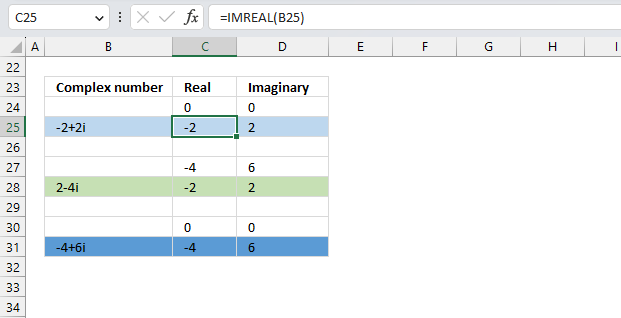Make sure that each complex number begins with real value 0 and imaginary value 0 on a separate row except the complex number you subtract with (green). The real and imaginary values on the next row, and that there is an empty row between. You can use the IMREAL function and the IMAGINARY function to extract the coordinates from the complex numbers in column B, make sure they are in rectangular form.

Formula in cell C25:

=IMREAL(B25)

Formula in cell D25:

=IMREAL(B25)

Copy these cells and paste to cells C28 and D28 respectively.

Formula in cell B31:

=IMSUB(B25, B28)

The row before complex number 2-4i (row 27) must contain the result from cells C31 and D31.

### 5.2 Insert scatter chart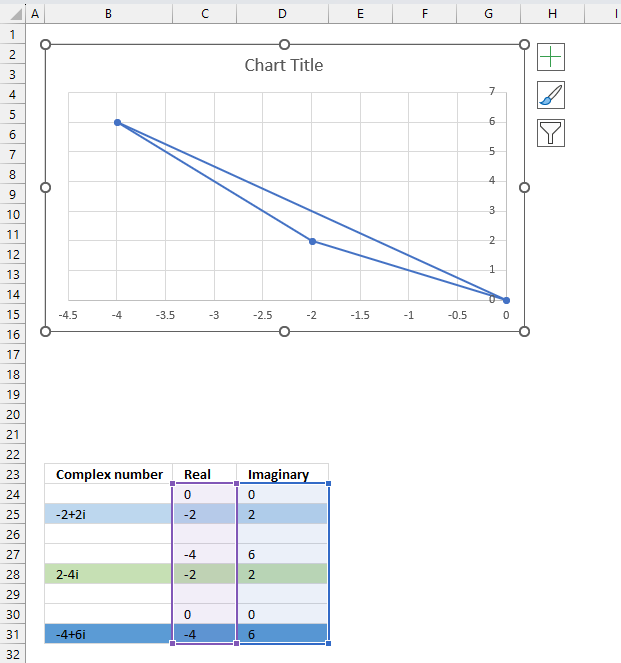1. Select cell range C24:D31.
2. Go to tab "Insert" on the ribbon.
3. Press with mouse on the icon named "Insert Scatter (x,y)", a popup menu appears.
4. Press with left mouse button on the icon "Scatter with Straight Lines and Markers".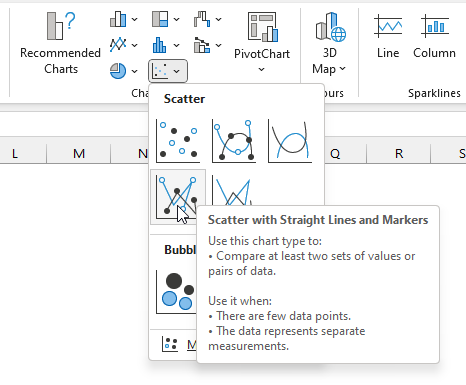5. The chart shows up on the worksheet.

Press and hold with left mouse button on the chart border, then drag it to the location you want. Use the "handles" to resize the chart, see the image below.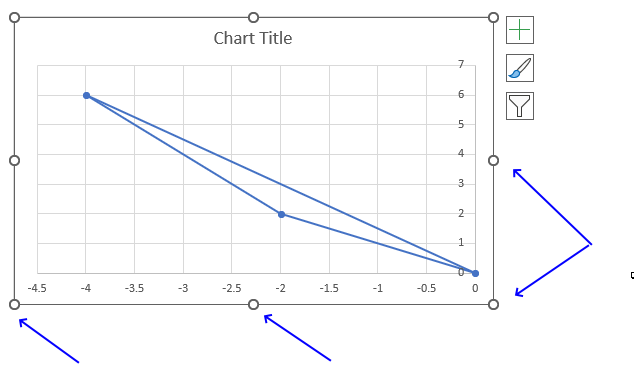### 5.3 Create arrows and change colors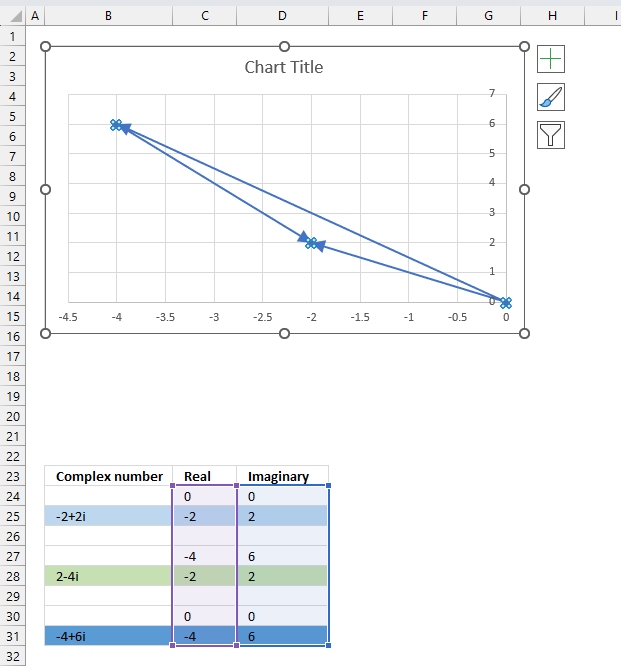1. Double-press with left mouse button on with left mouse button on one of the lines on the chart, all lines will be selected and a settings pane shows up.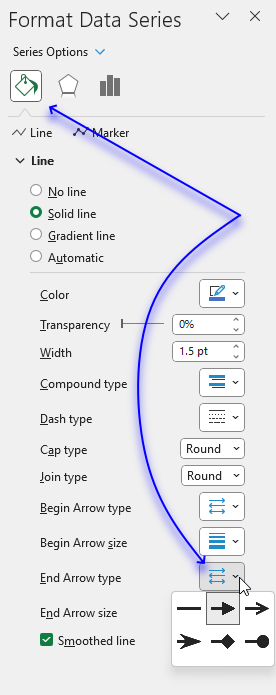2. Press with left mouse button on the "Fill&Line" button.
3. Press with mouse on "End Arrow type", select an arrow.
4. Press with mouse on "End Arrow size, pick a size.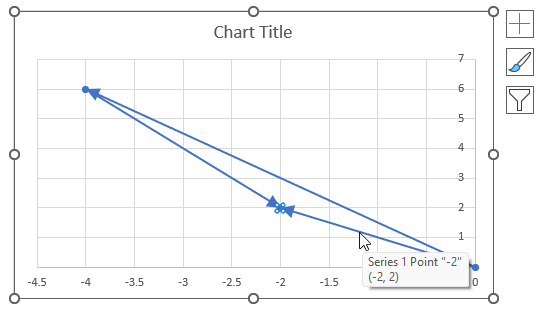1. Press with left mouse button on twice on a line to select a specific line.
2. Press with left mouse button on the color button on the settings pane. A popup menu appears.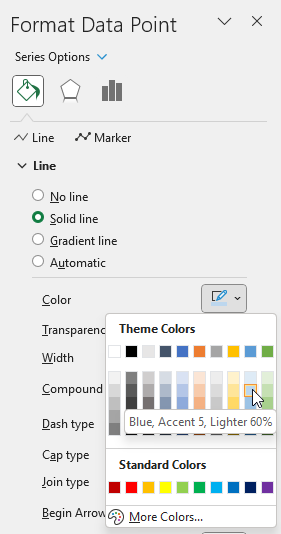3. Pick a color.
4. Repeat steps 1 to 3 with remaining lines.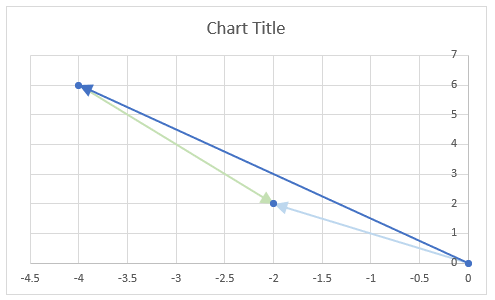There are still three markers on the chart, here is how to remove them.

1. Select all the lines by press with left mouse button oning on them once.
2. Go to the settings pane.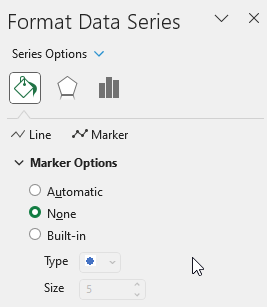3. Press with left mouse button on "Marker Options".
4. Select "None".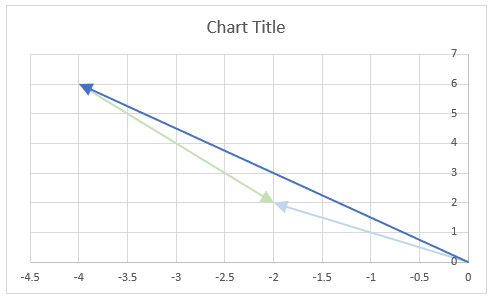### 5.4 Change axis min and max value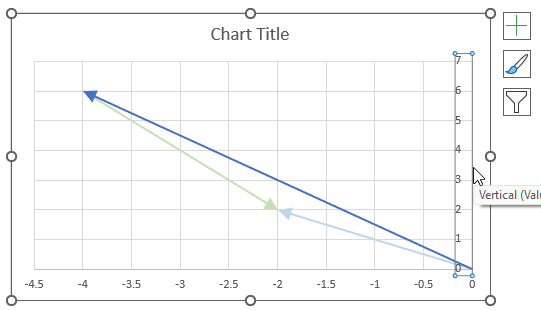1. Double-press with left mouse button on one of the y-axis to open the settings pane.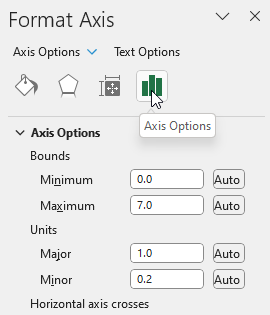2. Press with left mouse button on the "Axis Options" button.
3. Change the Bounds and the Units.
4. Repeat step 1 to 3 with the x-axis.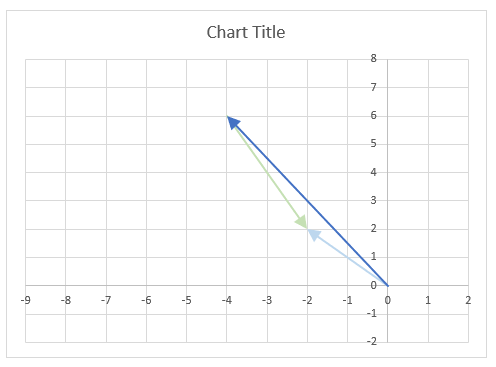### 5.5 Change axis markers and line width

1. Select the y-axis.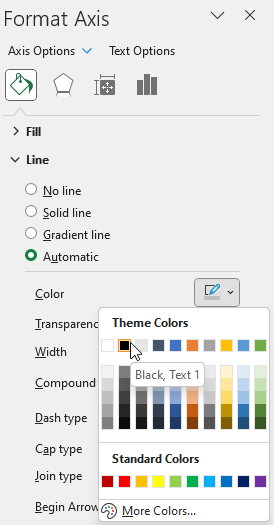2. Press with left mouse button on the "Fill & Line" button.
3. Press with left mouse button on the "Line".
4. Press with left mouse button on the "Color" button. Press with mouse on black.
5. Change the width to 1.5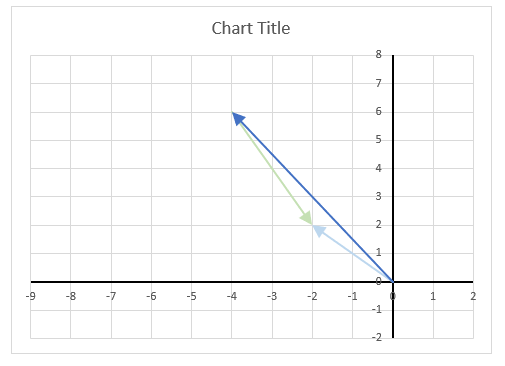1. Press with right mouse button on on one of the lines, a popup menu appears.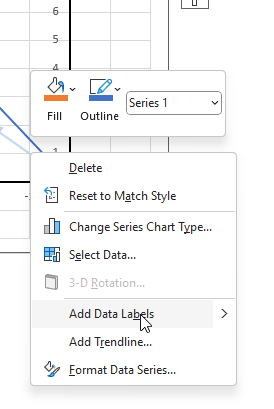2. Press with mouse on "Add Data Labels".
3. Double-press with left mouse button on one of the data labels, the settings pane shows up.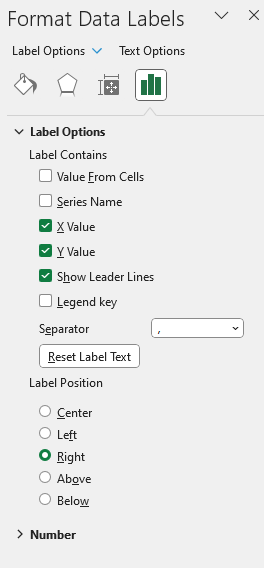4. Select "X Value" as well.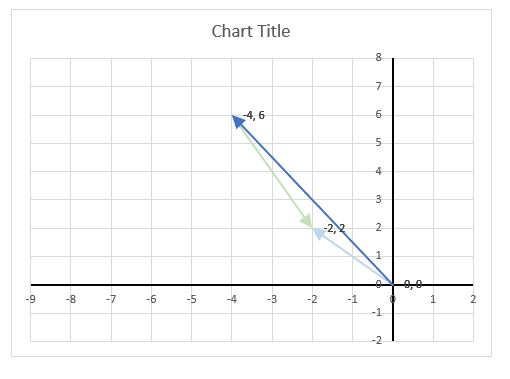Change the chart title and add axis titles.

## 6. How to calculate the distance between two complex numbers?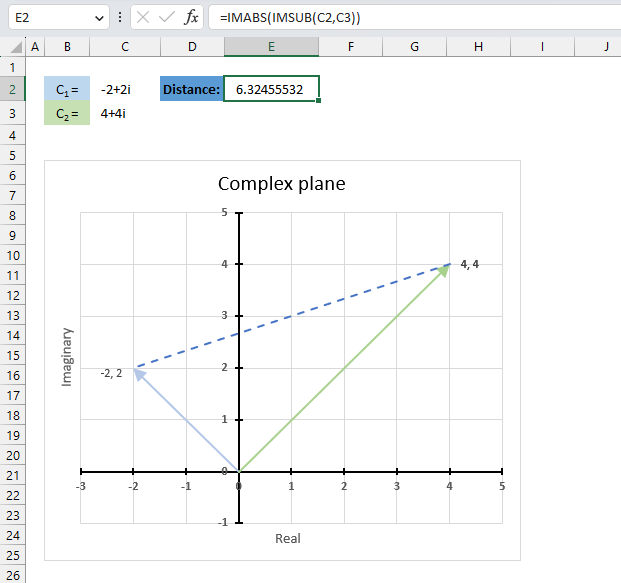The following formula calculates the length between two complex numbers in the complex plane. First, the formula subtracts the second complex number from the first, then it calculates the modulus of the difference.

For example, the first complex number is -2+2i and the second complex number is 4+4i. The difference is -6-2i, the modulus of -6-2i is the square root of 40 which equals approx. 6.32

Formula in cell E2:

=IMABS(IMSUB(C2,C3))

The image above shows the distance between these two points on the complex plane, the distance is the dashed blue line between -2+2i and 4+4i.

### Explaining formula in cell E2

#### Step 1 - Calculate the difference between two complex numbers

The IMSUB function calculates the difference between two complex numbers in x + yi or x + yj text format.

Function syntax: IMSUB(inumber1, inumber2)

IMSUB(C2,C3)

becomes

IMSUB("-2+2i","4+4i")

and returns

"-6-2i"

#### Step 2 - Calculate the modulus of a complex number

The IMABS function calculates the absolute value (modulus) of a complex number in x + yi or x + yj text format.

Function syntax: IMABS(inumber)

IMABS(IMSUB(C2,C3))

becomes

IMABS("-6-2i")

and returns

6.32